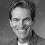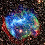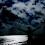## Thursday, April 2, 2015

### The sources of stock market fluctuations

How much do dividend-growth vs. discount-rate shocks account for stock price variations?

An under-appreciated point occurred to me while preparing for my Coursera class and to comment on Daniel Greewald, Martin Lettau and Sydney Ludvigsson's nice paper "Origin of Stock Market Fluctuations" at the last NBER EFG meeting

The answer is, it depends the horizon and the measure. 100% of the variance of price dividend ratios corresponds to expected return (discount rate) shocks, and none to dividend growth (cash flow) shocks.  50% of the variance of one-year returns corresponds to cashflow shocks. And 100% of long-run price variation corresponds to from cashflow shocks, not expected return shocks. These facts all coexist

I think there is some confusion on the point. If nothing else, this makes for a good problem set question.

The last point is easiest to see just with a plot. Prices and dividends are cointegrated. Prices correspond to dividends and expected returns. Dividends have a unit root, but expected returns are stationary. Over the long run prices will not deviate far from dividends. So 100% of long-enough run price variation must come from dividend variation, not expected returns.
Ok, a little more carefully, with equations.

A quick review:

The most basic VAR for asset returns is $\Delta d_{t+1} = b_d \times dp_{t}+\varepsilon_{t+1}^{d}$ $dp_{t+1} = \phi \times dp_{t} +\varepsilon_{t+1}^{dp}$ Using only dividend yields dp, dividend growth is basically unforecastable $$b_d \approx 0$$ and $$\phi\approx0.94$$ and the shocks are conveniently uncorrelated. The behavior of returns follows from the identity, that you need more dividends or a higher price to get a return,  $r_{t+1}\approx-\rho dp_{t+1}+dp_{t}+\Delta d_{t+1}%$ (This is the Campbell-Shiller return approximation, with $$\rho \approx 0.96$$.) Thus, the implied regression of returns on dividend yields, $r_{t+1} = b_r \times dp_{t}+\varepsilon_{t+1}^{r}$ has $$b_r = (1-\rho\phi)+0 = 1-0.96\times0.94 = 0.1$$ and a shock negatively correlated with dividend yield shocks and positively correlated with dividend growth shocks.

The impulse response function for this VAR naturally suggests "cashflow" (dividend) and "expected return" shocks, (d/p). (Sorry for recycling old points, but not everyone may know this.)

Three propositions:
• The variance of p/d is 100% risk premiums, 0% cashflow shocks
Iterate forward the return identity, to get $dp_{t} =\sum_{t=1}^{\infty}\rho^{j-1}r_{t+j}-\sum_{t=1}^{\infty}\rho ^{j-1}\Delta d_{t+j}$ multiply by $$dp_t$$ and take expectations (all variables are demeaned) $\sigma^{2}\left( \log\frac{P_{t}}{D_{t}}\right) =\sigma^{2}\left( dp_{t}\right) =\sum_{t=1}^{\infty}\rho^{j-1}cov(dp_{t},r_{t+j})-\sum _{t=1}^{\infty}\rho^{j-1}cov(dp_{t},\Delta d_{t+j}),$ But $$b_d \approx 0$$, so the dividend growth terms are all zero, and 100% of the variance of price-dividend ratios corresponds to time-varying expected returns. (I know this will bore people familiar with it and befuddle those who are not. "Discount rates" has a bit more leisurely review and citations)

But
•  The variance of returns is 50% due to risk premiums, 50% due to cashflows.
$r_{t+1}=-\rho dp_{t+1}+dp_{t}+\Delta d_{t+1}%$ $\varepsilon_{t+1}^{r} =-\rho\varepsilon_{t+1}^{dp}+\varepsilon_{t+1}^{d}$ $\sigma^{2}\left( \varepsilon_{t+1}^{r}\right) =\rho^{2}\sigma^{2}\left( \varepsilon_{t+1}^{dp}\right) +\sigma^{2}\left( \varepsilon_{t+1}^{d}\right)$ The variance of the two shocks comes out very close to a 50/50 decomposition at an annual horizon. It's a lot more expected return at a daily horizon, and less at longer horizons. Here I use the fact that dividend growth and dividend yield shocks are basically uncorrelated.

Why are returns and p/d so different?  Current cash flow shocks affect returns. But a shock to dividends, when prices rise at the same time, does not affect the dividend price ratio. (This is the essence of the Campbell-Ammer return decomposition.)

The third proposition is less familiar:
• The long-run variance of stock market values (and returns) is 100% due to cash flow shocks and none to expected return or discount rate shocks.
Here's why: $\Delta p_{t+1} =-dp_{t+1}+dp_{t}+\Delta d_{t+1}$ $p_{t+k}-p_{t} =-dp_{t+k}+dp_{t}+\sum_{j=1}^{k}\Delta d_{t+j}$ so as k gets big, ${var} (p_{t+k}-p_{t}) \rightarrow 2 {var}(dp_t) + k {var}(\Delta d_{t})$ The first term approaches a constant, but the second term keeps growing. As above the central fact is that P and D are cointegrated while expected returns are stationary.

This is related to a point made by Fama and French in their Equity Premium paper. Long run average returns are driven by long run dividend growth  plus the average value of the dividend yield. The difference in valuation -- higher prices for given set of dividends -- can affect returns in a sample, as higher prices for a given set of dividends boost returns. But that mechanism can't last. (Avdis and Wachter have a nice recent paper formalizing this point.)  It's related to a similar point made often by Bob Shiller: Long run investors should buy stocks for the dividends.

A little more generality as this is the new bit.

$p_{t+k}-p_t = dp_{t+k}-dp_t + \sum_{j=1}^{k}\Delta d _{t+j}$ $p_{t+k}-p_t = (\phi^{k}-1)dp_t + \sum_{j=1}^{k}\phi^{k-j} \varepsilon^{dp}_{t+j} + \sum_{j=1}^{k} \varepsilon^d _{t+j}$ $var(p_{t+k}-p_t) = \frac{(1-\phi^{k})^2}{1-\phi^2} \sigma^2(\varepsilon^{dp}) + \frac{(1-\phi^{2k})}{1-\phi^2} \sigma^2(\varepsilon^{dp}) + k\sigma^2(\varepsilon^d)$ $var(p_{t+k}-p_t) = 2\frac{(1-\phi^{k})}{1-\phi^2} var(\varepsilon^{dp}_{t+1}) + k var(\varepsilon^d_{t+j})$ So you can see the last bit takes over. It doesn't take over as fast as you might think. Here's a graph using sample values,

At a one year horizon, it's just about 50/50. The dividend shocks eventually take over, at rate 1/k. But at 50 years, it's still about 80/20.

Exercise for the interested reader/finance professor looking for problem set questions: Do the same thing for long horizon returns, $$r_{t+1}+r_{t+2}+...+r_{t+k}$$ using $$r_{t+1} = -\rho dp_{t+1} + dp_t + \Delta d_ {t+1}$$ It's not so pretty, but you can get a closed form expression here too, and again dividend shocks take over in the long run.

Be forewarned, the long run return has all sorts of pathological properties. But nobody holds assets forever, without eating some of the dividends.

Disclaimer: Notice I have tried to say "associated with" or "correspond to" and not "caused by" here! This is just about facts. The facts have just as easy a "behavioral" interpretation about fads and bubbles in prices as they do a "rationalist" interpretation. Exercise 2: Write the "behavioralist" and then "rationalist" introduction / interpretation of these facts. Hint: they reverse cause and effect about prices and expected returns, and whether people in the market have rational expectations about expected returns.

1.Your disclaimer at the end notwithstanding, I think it is worthwhile pointing out that your analysis does not rule out rational bubbles as a contributing factor in explaining variation i price-dividend ratios (a point that is often neglected or misunderstood).

phi = 0.94, b_d = 0, and b_r = 0.1 are fully consistent with a bubble, e.g. the partially and periodically bursting rational bubble in Evans (AER 1991). Cointegration between prices and dividends is also fully consistent with a bubble, in contrast to what is often claimed.

Lots of econometric evidence over the last 10 years points to explosive elements in price-dividend and price-earnings ratios, consistent with a rational bubble.

For details and further references, see Engsted, Pedersen and Tanggaard (JFQA 2012): http://journals.cambridge.org/action/displayAbstract?fromPage=online&aid=8675165&fileId=S0022109012000191 .

1.Actually "rational" bubbles, violations of the transversality condition, are testable. They imply a p/d ratio that grows forever in expectation. And the test fails (I spent a lot of my youth fascinated by these. Too bad.) More details in the first section of "discount rates" if you want to follow up.

The discussion here points out that these particular facts can't tell you if the variation in expected return -- high prices mean low expected returns -- is "rational," agents know about it and it reflects changes in risk aversion, or "irrational", agents think otherwise and we're picking up the true expected returns that only the Wise Academics know is what's really going on.

2.The Evans (AER 1991) bubble model does not imply a p/d ratio that grows forever. And the analysis in the first section of "Discount Rates" does not rule out bubbles. phi = 0.94, b_d = 0, and b_r = 0.1 are fully consistent with a bubble, see Engsted, Pedersen and Tanggaard (JFQA, 2012).

2.Off, topic, but, SImon Wren Lewis has an interesting new post, you can take a look if you want.
http://mainlymacro.blogspot.com/2015/04/do-not-underestimate-power-of.html

3.Question from: "The Fiscal Theory of the Price Level
and its Implications for Current Policy in the United States and Europe", the basic equation only includes flows in order to solve for a stock, value of the debt at time t, but what about another stock, net assets of the sovereign? In particular, could this one-time-tax be interpreted as a sale of sovereign assets? Thanks for any reply.
"...“Fiscal theory” then, just amounts to paying a bit more attention to where the fiscal part of coordination
comes from, and whether it is in place. If you’re examining a gold standard, you want to know if the
country has taxing power to obtain or borrow gold, or to avoid raiding its reserves. “Fiscal theory”
means making a similar examination of today’s monetary‐fiscal coordination.
All monetary models contain a (sometimes implicit) Ricardian footnote, “the Treasury passively adjusts
lump‐sum taxes to pay off changes in the real value of government debt.”..."

1.you can add real assets as either the flow of surpluses they generate, or add their value. B/P - b (real assets) = E sum ...

2.Greatly obliged to you, prof. Cochrane, and I think I understand this point, I was just trying to reconcile applied finance (my field) and theory, to wit: "....All monetary models contain a (sometimes implicit) Ricardian footnote, “the Treasury passively adjusts
lump‐sum taxes to pay off changes in the real value of government debt.”..."..."

If a sovereign sells off non-income-producing assets (unencumbered state lands, say) in a micro-sense it's like me discovering I owe lots more money than I have and doing an inventory of my silverware, jewelry, fur coats, cars, etc. But in a macro sense, as in eg Greece, could this monetization of non-productive-assets, be viewed as mathematically identical to the Ricardian footnote in your text?

4.Perhaps, an example from US debt, in this book:
https://ficpress.wordpress.com/2012/11/28/is-u-s-government-debt-different/
Chapter 12, titled Burning the Furniture to Heat the House – The Potential Role of Asset
Sales in Funding the Federal Government’s Deficits by Jim Millstein.

"......The aggregate sales price of all of these non-defense assets could be
between $4.3 and$5.4 trillion, which, if applied to debt reduction,
could decrease publicly-held debt as a share of GDP to between 36
and 43 percent—well below the 90 percent threshold associated with
slower growth26 and in line with AAA-rated Sweden......"

5.Final comment on asset sales, and on Greece:
http://www.rolandberger.de/medien/news/2012-01-31-rbsc-news-EURECA_in_2012.html
Failed to avert 2 bailouts because of grotesque scale of fraud, waste, abuse in Greek national accounts – nobody will buy assets without due diligence without an audit. This fraud is contagious, as evident in following quote from James Galbraith:
http://www.newyorker.com/magazine/2015/03/09/greeces-next-move
“…Galbraith said, “When they went to the Ministry of Finance for the first time, there was not a document, not a computer. The Wi-Fi was not turned on.”..”
But the employees were there, right? Must have been, as headcount and personnel costs at the Ministry of Finance both increased since Syriza formed a government – so how about someone asks those employees, do they know anything about the missing documents and equipment? If not, why not?

6.The math is beyond me, but how does this take into account other means of returning cash to shareholders (ie. buybacks)

7.Prices and dividends are cointegrated; therefore no persistent variation in expected returns. What about secular stagnation? Does that not imply real yields have permanently dropped to 0 or below? How can that not show up as an I(1) error in the cointegrating regression?

8.Prof Cochrane,

Apologies for coming to this so late; this is a wonderful model to view prices through.

The view that earnings and prices are co-integrated seems to give rise to the following two interpretations:

- there is a long term price change driven by the cashflow/fundamentals of the stock market and a short term volatility driver that contributes little to the long term price change. This means that there is a drift component that should show up in short term returns that is just a function of this "excess short term" volatility (0=Mu - 0.5* Variance or Mu = 0.5* Variance for a lognormal return process) even if there is no change in prices.

- you can construct a portfolio over the term 'h' that is a sum of the buy and hold "t+h" returns and one that is short this "short term" volatility. I am interpreting this as rebalancing return based on your idea that rebalancing is akin to being short volatility; and it seems the math is exploiting not the volatility itself but the "diversification effect" of Fama-Booth. That formulation is independent of distributional assumptions and so can take higher moments. The first component will be driven by a different driver of variance (dividend in your formulation) while the second component will be driven by another (expected return in your read). And if the long term buy and hold returns are a function of cashflows- we might be better off looking at industrial economics based ideas to earn long term returns (strategic competitive advantage, rents earned) like the Value investors do.

A second possible interpretation is that long term co-integration leads to a error correction model over the short run which if we mine for "largest" mean reversion looks a lot like the "Value portfolio" of Fama-French (long stocks that have the smallest "log price-log earnings" and vice versa). Or can we legitimately see Value factor returns as just this short term volatility induced by co-integration between prices and fundamentals like book value or earnings or dividends or cash flows showing up as "expected returns".

If this is plain wrong, do let me know. And if there is academic work that resembles this, I would appreciate your directing me to them.

Sunil

Comments are welcome. Keep it short, polite, and on topic.

Thanks to a few abusers I am now moderating comments. I welcome thoughtful disagreement. I will block comments with insulting or abusive language. I'm also blocking totally inane comments. Try to make some sense. I am much more likely to allow critical comments if you have the honesty and courage to use your real name.